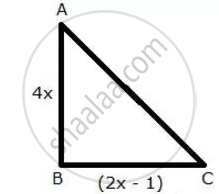Share

The Sides of a Right-angled Triangle Containing the Right Angle Are 4x Cm and (2x – 1) Cm. If the Area of the Triangle is 30 Cm²; Calculate the Lengths of Its Sides. - ICSE Class 10 - Mathematics

Question

The sides of a right-angled triangle containing the right angle are 4x cm and (2x – 1) cm. If the area of the triangle is 30 cm²; calculate the lengths of its sides.

SolutionArea of triangle = 30 cm2

∴ 1/2 xx (4x) xx (2x - 1) = 30

2x^2 - x = 15

2x^2 - x - 15 = 0

2x^2 - 6x + 5x - 15 = 0

2x(x - 3) + 5(x - 3) = 0

(x - 3)(2x + 5) = 0

x = 3, (-5)/2

But x cannot be negative so x= 3

Thus we have

AB = 4 x 3 cm  = 12 cm

BC = (2 x  3 - 1) cm = 5 cm

CA = sqrt(12^2 + 5^2) cm  = 13  cm (Using Pythagoras therorem)

Is there an error in this question or solution?

APPEARS IN

Selina Solution for Selina ICSE Concise Mathematics for Class 10 (2018-2019) (2017 to Current)
Chapter 6: Solving (simple) Problems (Based on Quadratic Equations)
Ex.6B | Q: 1

Video TutorialsVIEW ALL 

Solution The Sides of a Right-angled Triangle Containing the Right Angle Are 4x Cm and (2x – 1) Cm. If the Area of the Triangle is 30 Cm²; Calculate the Lengths of Its Sides. Concept: Quadratic Equations.
S